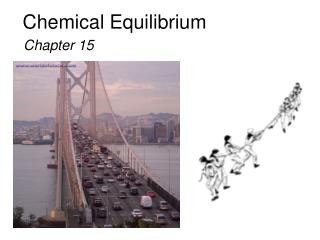DownloadDownload PresentationChemical Equilibrium

# Chemical Equilibrium

Download Presentation## Chemical Equilibrium

- - - - - - - - - - - - - - - - - - - - - - - - - - - E N D - - - - - - - - - - - - - - - - - - - - - - - - - - -
##### Presentation Transcript

1. Chemical Equilibrium Chapter 15

2. Chemical Equilibrium A state of balance dynamic static

3. Chemical Equilibrium A state of balance Static- two sides pulling with equal force results in an object at rest Dynamic- rate of cars leaving equals rate of cars entering so no net change in the number of cars in the city Other examples of dynamic equilibrium include vapor in equilibrium with liquid and solid in equilibrium with liquid. Chemical- opposing reactions are proceeding at equal rates and there is no net change in the concentrations of reactants and products.

4. H2O (l) H2O (g) N2O4(g) 2NO2(g) Equilibrium is a state in which there are no observable changes as time goes by. • Chemical equilibrium is achieved when: • the rates of the forward and reverse reactions are equal and • the concentrations of the reactants and products remain constant Physical equilibrium Chemical equilibrium 15.1

5. N2O4(g) 2NO2(g) • No net change in concentrations of reactants and products • Forward and reverse rates are equal • Reaction has NOT stopped • For equilibrium to occur, neither reactants nor products can escape from the system • At equilibrium, a particular ratio of the concentration terms equals a constant

6. [NO2]2 [N2O4] kf kr = =Keq N2O4(g) 2NO2(g) • Forward reaction: N2O4 (g) 2 NO2 (g) • Rate law: Rate = kf [N2O4] • Reverse reaction: 2 NO2 (g) N2O4(g) • Rate law: Rate = kr [NO2]2 Therefore, at equilibrium Ratef = Rater kf [N2O4] = kr [NO2]2 Rewriting this, it becomes

8. constant 15.1

9. N2O4(g) 2NO2(g) K = aA + bB cC + dD [NO2]2 [C]c[D]d [N2O4] K = [A]a[B]b = 4.63 x 10-3 Law of Mass Action Equilibrium Will K >> 1 Lie to the right Favor products K << 1 Lie to the left Favor reactants 15.1

10. N2O4(g) 2NO2(g) Kc = Kp = In most cases Kc Kp P2 [NO2]2 NO2 [N2O4] aA (g) + bB (g)cC (g) + dD (g) P N2O4 Homogenous equilibrium applies to reactions in which all reacting species are in the same phase. Kp = Kc(RT)Dn Dn = moles of gaseous products – moles of gaseous reactants = (c + d) – (a + b) 15.2

11. CH3COOH (aq) + H2O (l) CH3COO-(aq) + H3O+ (aq) ‘ ‘ Kc = Kc [H2O] General practice not to include units for the equilibrium constant. [CH3COO-][H3O+] [CH3COO-][H3O+] = [CH3COOH][H2O] [CH3COOH] Homogeneous Equilibrium [H2O] = constant Kc = 15.2

12. The equilibrium concentrations for the reaction between carbon monoxide and molecular chlorine to form COCl2 (g) at 740C are [CO] = 0.012 M, [Cl2] = 0.054 M, and [COCl2] = 0.14 M. Calculate the equilibrium constants Kc and Kp. CO (g) + Cl2(g) COCl2(g) = 0.14 0.012 x 0.054 [COCl2] [CO][Cl2] = 220 Kc= Kp = Kc(RT)Dn Dn = 1 – 2 = -1 R = 0.0821 T = 273 + 74 = 347 K Kp= 220 x (0.0821 x 347)-1 = 7.7 15.2

13. The equilibrium constant Kp for the reaction is 158 at 1000K. What is the equilibrium pressure of O2 if the PNO = 0.400 atm and PNO = 0.270 atm? 2 PNO PO = Kp 2 PNO 2 Kp = 2NO2 (g) 2NO (g) + O2 (g) PO PO = 158 x (0.400)2/(0.270)2 2 2 2 2 PNO PNO 2 2 2 = 347 atm 15.2

14. CaCO3(s) CaO (s) + CO2(g) ‘ ‘ Kc = Kc x [CaO][CO2] [CaCO3] [CaCO3] [CaO] Kp = PCO 2 Heterogenous equilibrium applies to reactions in which reactants and products are in different phases. [CaCO3] = constant [CaO] = constant Kc= [CO2] = The concentration of solids and pure liquids are not included in the expression for the equilibrium constant. 15.2

15. CaCO3(s) CaO (s) + CO2(g) PCO PCO 2 2 does not depend on the amount of CaCO3 or CaO = Kp 15.2

16. Consider the following equilibrium at 295 K: The partial pressure of each gas is 0.265 atm. Calculate Kp and Kcfor the reaction? Kp = P NH3 NH4HS (s) NH3(g) + H2S (g) P H2S = 0.265 x 0.265 = 0.0702 Kp = Kc(RT)Dn Kc= Kp(RT)-Dn Dn = 2 – 0 = 2 T = 295 K Kc = 0.0702 x (0.0821 x 295)-2 = 1.20 x 10-4 15.2

17. A + B C + D C + D E + F A + B E + F [C][D] [E][F] [E][F] [C][D] [A][B] [A][B] Kc = ‘ ‘ ‘ Kc Kc Kc = If a reaction can be expressed as the sum of two or more reactions, the equilibrium constant for the overall reaction is given by the product of the equilibrium constants of the individual reactions. ‘ ‘ Kc = x Kc = ‘ ‘ ‘ ‘ Kc Kc Kc 15.2

18. N2O4(g) 2NO2(g) 2NO2(g) N2O4(g) ‘ K = K = = 4.63 x 10-3 When the equation for a reversible reaction is written in the opposite direction, the equilibrium constant becomes the reciprocal of the original equilibrium constant. [NO2]2 [N2O4] [N2O4] [NO2]2 1 K = = 216 15.2